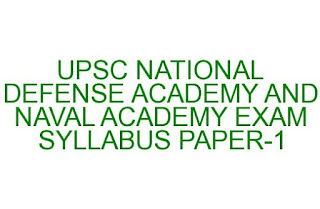# E Admit Card UPSC NDA AND NA EXAM SYLLABUS PATTERN PAPER 1 2022-Exam Date 10-04-2022

## UPSC NDA AND NA EXAM SYLLABUS PATTERN 2022-ADMIT CARD

UPSC NDA and NA Exam Pattern Syllabus 2022: Union Public Service Commission of India has been invited applications for the Graduate Level Program for NDA and NA officers from the citizens of India. The last date to apply online is 10-04-2022. This is the first phase of UPSC NDA and NA Examination. For the second phase notification will be released on July or August months.  There are 400 vacancies available which are 370 for National Defense Academy and 30 for Naval Academy. The exam will be conducted in two stages followed by a personal interview. Paper 1 examination for 300 marks with two and half hours time duration and Paper 2 exam will be for 600 marks with the same duration followed by a SSB interview for 900 marks total marks for UPSC NDA and NA Examination 2022 is 1800 marks. Based on all these marks final list will be generated. Here we will see the complete syllabus and pattern of UPSC NDA and NA examination.

## UPSC Naval Academy and National Defense Academy Exam Syllabus and Pattern 2022

#### UPSC NDA and NA exam Syllabus for Paper-I:

Paper-I is purely based on Mathematics. Paper-I consist of 300 marks in UPSC NDA and NA Exam.

####1. Algebra concepts for UPSC NDA and NA Exam in Paper I:

Logs and its applications, Binomial theorem, P&C, Linear In equations, Quadratic
equations, AM,GM and HM progressions, Decimal to binary conversions, Complex numbers, Real Numbers, Cartesian product, equivalence relation, relation, operations on sets, Concept of set, , Venn diagrams, De Morgan laws.

#### 2. Matrices and Determinants concepts of Paper I NDA & NA Exam 2022:

Operations of Matrices, Types of Matrices, on matrices, basic properties of determinants, Determinant of a matrix, Adjoint, inverse of a square matrix, Cramer’s rule.

#### 3. UPSC NDA and NA Exam 2022 in Paper I concepts ofTRIGONOMETRY:

Angles, Trigonometric identities, Inverse trigonometric functions, properties of triangles, radians, Trigonometrical ratios, Trigonometric identities, Multiple, Sub-multiple angles, Applications-Height and distance.
4. Analytical Geometry Of Two And Three Dimensions:
Equation of a sphere, Distance formula, Rectangular Cartesian Coordinate system, Various forms of  Equation of a line, Distance from a point to a line, Angle between two lines, Circle equation in standard and in general form, ellipse and hyperbola,  Parabola Standard forms, Eccentricity, distance between two points , axis of a conic, three dimensional space of point, Direction ratios and direction Cosines,  Equation two points, various forms of a plane and line of a equation, Angle between two Planes and
Two lines, Equation of a plane and a line in various forms.
5. Differential Calculus concepts of 2022 UPSC NA and NDA Paper I Exam:
Topics of a real valued function – range, domain and function graph, one to one, Composite functions, Notion of limit, onto and inverse functions, examples of standard limits, examples of Continuity functions, algebraic operations, At a point derivative of function, Derivatives of sum, physical interpretation and geometrical of a derivative and its applications, quotient and product of functions, Second order derivatives, composite function derivatives, decreasing and increasing functions, Maxima and minima Application of Derivatives in sums.
6. UPSC NDA Paper 1 Syllabus Integral Calculus and Differential Equations:
Differentiation of inverse integration, integration by parts and by substitution, algebraic expressions with standard integrals, exponential, trigonometric, hyperbolic functions, definite integrals—determination of curves-applications, Definition of degree and order of a differential equation, formation of a D.E. by examples. General, particular solution of a D.E., Application in problems of decay
and growth, solution of first order, degree differential, various types equations and its examples.
7. 2016 UPSC NDA and NA Paper I Exam concepts of Vector Algebra:
Vectors in 2 and 3 dimensions, magnitude, direction of a vector, Null and Unit vectors, vector addition, scalar multiplication of a vector, dot product or scalar product of 2 vector, Cross product or Vector product of 2 vectors, Applications of a geometrical problems and force.
8. Statistics and Probability:
Statistics: Data Classification, Frequency distribution, Distribution of cumulative, frequency and its examples. Graphical representation of Pie Chart, Histogram, frequency polygon and their examples, Concepts of Measures of Central tendency such as Median, Mean and mode, Correlation and regression, standard and Variance deviation of determination and comparison.
Probability: events, outcomes, associated sample space, Random experiment, exhaustive events and
Mutually exclusive, impossible and certain events, Intersection and Union of events, Complementary, elementary, composite events, Probability Definition and classical, statistical examples, Conditional probability, Probability Elementary theorems and simple problems, Bayes’ theorem and simple problems, Random variables, Binomial distribution and examples of random experiments supporting  Binominal distribution.